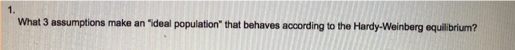# Problem: What 3 assumptions make an "deal population" that behaves according to the Hardy-Weinberg equilibrium?

###### FREE Expert Solution

The 3 assumptions that makes the ideal population according to the Hardy Weinberg equilibrium are as follows:

• All individuals have equal rates of survival and equal reproductive success, thus no natural selection occur.###### Problem Details

What 3 assumptions make an "deal population" that behaves according to the Hardy-Weinberg equilibrium?